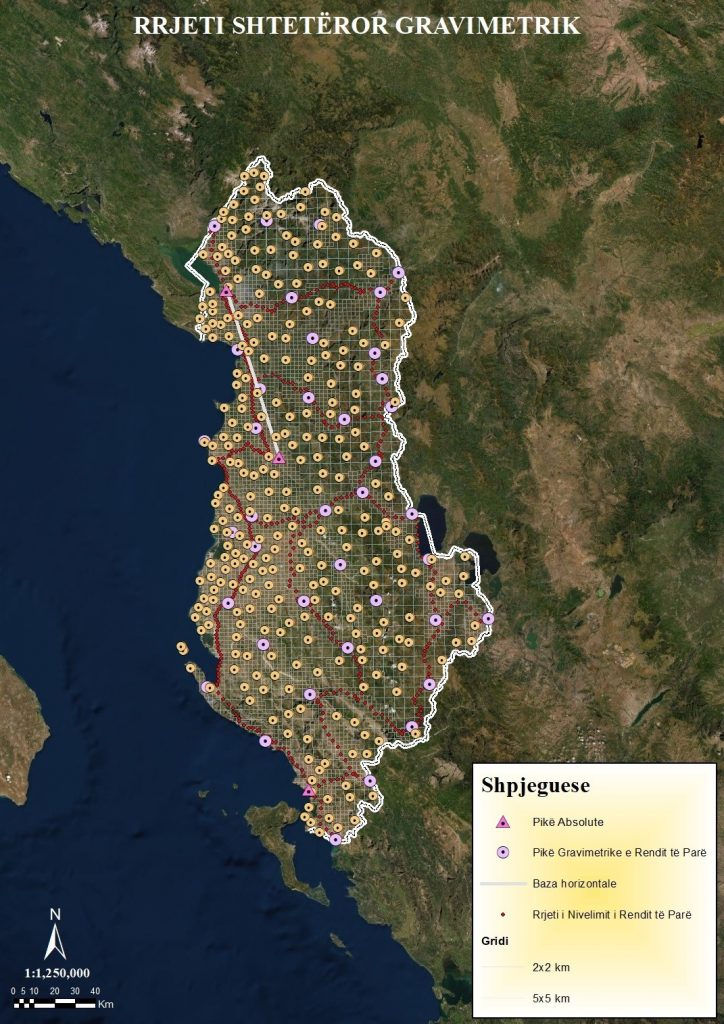# Gravimetric NetworkThe main goal of the State Gravimetric Network is to provide a qualitative database of gravimetric data needed to determine the high-precision gravimetric geoid for the territory of Albania, which will serve to build a new system of physical heights in accordance with European standards.

The State Gravimetric Network is part of the KRGJSH and has in its composition:

1. Zero State Gravimetric Network;
2. State Grammar Network of the First Order;
3. Gravimetric Network of the Second Order;
4. Gravimetric Network of the Third Order;

The absolute gravimetric points of the zero order (Shkodra, Tirana, Saranda) and the 42 points of the relative gravimetric network of the first order constitute the Albanian Gravimetric Datum.

The unit of measurement for gravitational acceleration of gravity is ms-2 (meter per second per square).

### Zero Order Gravimetric Network

The State Gravimetric Network of Zero Order consists of three stations in which the absolute value of the gravitational acceleration of gravity is measured using absolute gravimeters of high accuracy. Absolute stations in the territory of the country are those of Shkodra, Tirana and Saranda.

The Zero State Gravimetric Network serves as a basis for supporting gravimetric networks of the lowest order and enables the preservation of the Albanian Gravimetric Datum by performing measurements once every 10 years at these points.

Absolute gravimetric points are stabilized in massive concrete blocks built on stable geological structures in accordance with international recommendations.

### Gravimetric Network of the First Order

The State Gravimetric Network of the First Order is part of the Albanian Gravimetric Datum and serves to thicken the State Gravimetric Network of the Zero Order.

The State Gravimetric Network of the First Order consists of 42 points uniformly distributed in the territory of the country at a distance of 35-50 km which are stabilized in concrete blocks built on stable geological structures in accordance with international recommendations.

The points of the State Gravimetric Network of the First Order are determined by the gravitational acceleration value of the gravitational force every 20 years using simultaneously at least two relatively calibrated gravimeters that provide high precision in measurements with relative gravimetric methods.

The standard deviation in determining the absolute acceleration of gravity at the points of the State Gravimetric Network of the First Order should not exceed the value 10 x 10-8 ms-2.

### Gravimetric Network of the Second Order

The Gravimetric Network of the Second Order serves for the further densification of the State Gravimetric Network of the First Order and consists of 38 points distributed in the territory of the country stabilized in concrete blocks.

The points of the Gravimetric Network of the Second Order are determined by the gravitational acceleration value of the gravitational force with relatively calibrated relative gravimeters that provide high precision in measurements with relative gravimetric methods.

The standard deviation in determining the absolute acceleration of gravity at the points of the Gravimetric Network of the Second Order should not exceed the value 20 x 10-8 ms-2.

### Gravimetric Network of the Third Order

The Gravimetric Network of the Third Order is the final densification of the State Gravimetric Network which enables the ground gravimetric data necessary for the calculation of the exact gravimetric geoid for the territory of the country, ALB Geoid 20XX.

The Gravimetric Network of the Third Order has the shape of a density grid:

• For plain areas, 1 point for every 2 km2;
• For mountainous areas, 1 point for every 5 km2;

The points of the Gravimetric Network of the Third Order are not stabilized in the field, their calculations and ellipsoidal heights are calculated based on the ALBCORS system.

The standard deviation in determining the absolute acceleration of gravity at the points of the Gravimetric Network of the Third Order should not exceed the value 30 x 10-8 ms-2.